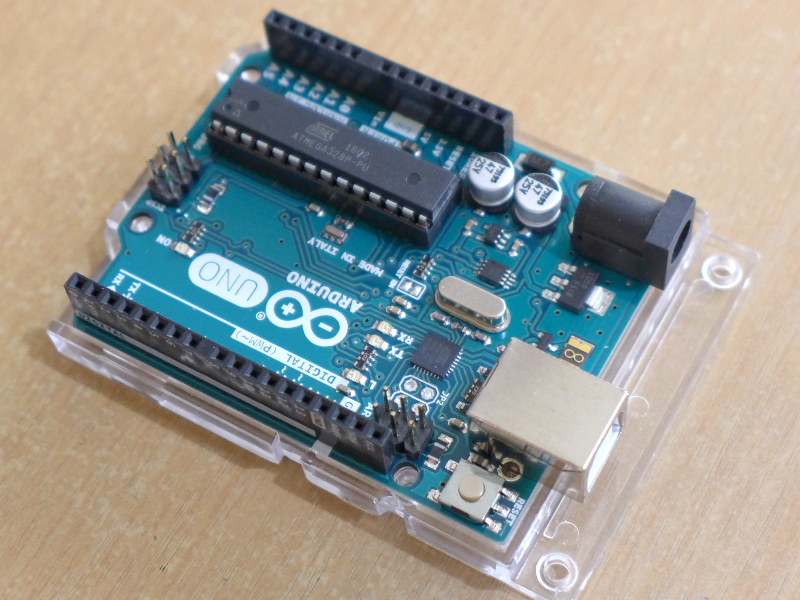# Arduino MapFloat Library Documentation

I have always used the Arduino IDE map function but have not been able to use it for float values. With these I have made the MapFloat Library so that I could extend the map function to floating point numbers.

A very useful feature when I want to have decimal places on my map function.

## Introduction

A library that contains the mapFloat function which re-maps a floating point number from one range to another. The mapFloat function is inside the MapFloat library.

This library is based on the Arduino Map Function.

Here is a link to the reference of Arduino Map Function.
https://www.arduino.cc/reference/en/language/functions/math/map/

## Installing the MapFloat Library

Unzip the MapFloat-master folder inside the zip file and paste it on the library folder where your Arduino IDE is installed.

Rename MapFloat-master folder to MapFloat only.

## Using the mapFloat function

Once you have installed the MapFloat Library you may now use the mapFloat function on your code as long as you add #include “MapFloat.h” at the top of your code.

Note: the MapFloat Library only contains a function. No instantiation of a class needed.

``y = mapFloat(x, 12.345, 89.67, 1000.23, 2512.45);``

The mapFloat function also acts like the Arduino map function but can handle floating point numbers.

Function name: mapFloat

Parameters:

• value (float)
• fromLow (float)
• fromHigh (float)
• toLow (float)
• toHigh (float)

Return: float – re-mapped value.

## Example

This .ino file can be accessed in Arduino via File >> Examples >> MapFloat >> MapFloatExample.

``````#include "MapFloat.h"

void setup() {
Serial.begin(115200);   // Initialize Serial
while(!Serial);         // Wait for Serial connection
}

void loop() {
float input, output;

input = 32.5;
output = mapFloat(input, 0.0, 100.0, 0.0, 1000.0);
Serial.println(input);
Serial.println(output);
Serial.println("++++");

input = 45.67;
output = mapFloat(input, 0.0, 100.0, 0.0, -1000.0);
Serial.println(input);
Serial.println(output);
Serial.println("++++");

input = 321.83;
output = mapFloat(input, 0.0, 1000.0, 1000.0, 2000.0);
Serial.println(input);
Serial.println(output);
Serial.println("++++");

input = 563.09;
output = mapFloat(input, 0.0, 1000.0, -1000.0, -2000.0);
Serial.println(input);
Serial.println(output);
Serial.println("++++");

input = 743.88;
output = mapFloat(input, 32.76, 1023.32, 3212.32, -5212.978);
Serial.println(input);
Serial.println(output);
Serial.println("++++");

while(true);
}``````

I hope this Library helps on your projects.

If there are any errors, questions or suggestions that you can give me about the mapFloat library kindly comment below or send me a message via our contact page.

## 5 thoughts on “Arduino MapFloat Library Documentation”

1.miguel cruz says:

muchasssss graciassssssss ¡¡¡

1.Radish Logic says:

You’re welcome!

2.Martynas says:

You solved all my problems

1.Radish Logic says:

I’m very glad to have helped Martynas! ^_^

3.djim says:

thanks

This site uses Akismet to reduce spam. Learn how your comment data is processed.